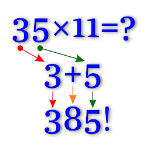# Math Tricks APK

Math Tricks

## Math Tricks description:

This program is designed for those who want to learn interesting mathematical tricks to speed up the calculating. These tricks will help solve part of the mathematical problems and tasks much faster than classical. Will also be helpful to those who want to hone basics such as the multiplication table.

When you learn these mathematical tricks, you'll be able to show off your skills to friends and prove to them that you have a talent for mathematics. New skills you can use in the store, at school, at college, at work - wherever thanks to quick calculation skills can save a lot of precious time.

Fun Math Tricks:
2. Subtraction
3. Multiplication table
4. Division
5. Multiply two digit number by 11
6. Square numbers ending in 5
7. Multiply by 5
8. Multiply by 9
9. Multiply by 4
10. Dividing by 5
11. Subtracting from 1000
12. Tough multiplication
13. Power of two
14. Adding numbers close to hundreds
15. Subtracting numbers close to hundreds
16. Multiply numbers between 11 and 19
17. Square numbers between 11 and 99
18. Multiply two digit numbers having same tens digit and ones digits add up to ten
19. Square numbers between 50 and 59
20. Square numbers between 40 and 49
21. Multiply two digit numbers editing in 1
22. Percent
23. Square numbers between 100 and 109
...
84. n^9

Training mode:
- 15 levels for every trick
- timer 15 seconds
Rating stars:
- score > 000 - ★☆☆
- score > 350 - ★★☆
- score > 650 - ★★★

The app uses the following APIs and libraries:
* MPAndroidChart - created by Philipp Jahoda
* ColorPIcker - created by Piotr Adamus
Older version of Math Tricks APK
Math Tricks 2.30 for Android 4.0 APK file

Version: 2.30 for Android Android 4.0

Update on: October 25 19

Size: 8.35 MB (8,754,943 bytes)

Math Tricks 2.29 for Android 4.0 APK file
Math Tricks 2.28 for Android 4.0 APK file
Math Tricks 2.27 for Android 4.0 APK file
Math Tricks 2.24 for Android 4.0 APK file
Math Tricks 2.23 for Android 4.0 APK file
Math Tricks 2.22 for Android 4.0 APK file
Math Tricks 2.21 for Android 4.0 APK file
Math Tricks 2.20 for Android 4.0 APK file
Math Tricks 2.19 for Android 4.0 APK file
Math Tricks 2.18 for Android 4.0 APK file
Math Tricks 2.17 for Android 2.3 APK file
Math Tricks 2.13 for Android 2.3 APK file
Math Tricks 2.12 for Android 2.3 APK file
Math Tricks 2.11 for Android 2.3 APK file
Math Tricks 2.10 for Android 2.3 APK file
Math Tricks 2.9 for Android 2.3 APK file
Math Tricks 2.7 for Android 2.3 APK file
Math Tricks 2.6 for Android 2.3 APK file
Math Tricks 2.4 for Android 2.3 APK file
Math Tricks 2.3 for Android 2.3 APK file
Math Tricks 2.2 for Android 2.3 APK file
Math Tricks 2.1 for Android 2.3 APK file
Math Tricks 2.0 for Android 2.3 APK file
Math Tricks 110 for Android 2.3 APK file
Math Tricks 111 for Android 2.3 APK file
Math Tricks 109 for Android 2.3 APK file
Math Tricks 97 for Android 2.3 APK file
Math Tricks 95 for Android 2.3 APK file
Math Tricks 108 for Android 2.3 APK file
Math Tricks 107 for Android 2.3 APK file
Math Tricks 106 for Android 2.3 APK file
Math Tricks 105 for Android 2.3 APK file
Math Tricks 104 for Android 2.3 APK file
Math Tricks 102 for Android 2.3 APK file
Math Tricks 101 for Android 2.3 APK file
Math Tricks 94 for Android 2.3 APK file
Math Tricks 96 for Android 2.3 APK file
User reviews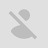Patrick Vp

Great math learning app. Very useful, but not everyone is good at this game. It takes time to learn effectively.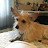Gugliaume Gundelinde

Timer ruins it. Not a helpful game. The game tells you the answer if you don't solve the problem fast enough. The only way to get better is if you solve the problems on your own. I dont have very fast mental math skills and want to improve, but being given the answer takes out all the work and any chance of improvement. The settings will not allow you to turn the timer off. The timer is what makes this game worthless, in my opinion. I hope they change it in a later version, but in the meantime I'm deleting it and looking for something else.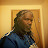Brian M. Mc Washington

It's tough at first, but it tells you how to get the answer in a simple way.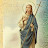Sky Cloud

Very good Unlike most math apps, this one makes math work for you.Jan Cosgrove

Like a brain training exercise learning as well never been great at maths but it's all explained clearly still trying to get my head round square numbers but slowly understanding